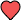# TI-89 Titanium

## Getting Started

The TI-89 Titanium is a powerful graphing calculator that makes advanced calculations and 3D graphing easier for engineering and science courses. There is a built-in Computer Algebra System (CAS) that allows you to explore and manipulate mathematical expressions in symbolic form.

• Operations |
• # |
• A |
• B |
• C |
• D |
• E |
• F |
• G |
• H |
• I |
• J |
• K |
• L |
• M |
• N |
• O |
• P |
• Q |
• R |
• S |
• T |
• U |
• V |
• W |
• X |
• Y |
• Z |
• See all

Operations

No terms.

## Programming

Mid-Point Formula

Formula

Formula© 2019 Infinity Calcs | Made with